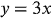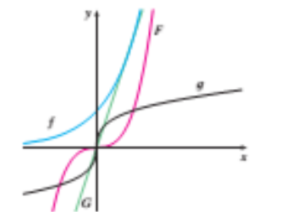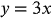1
53
views
4a
Problem

Problem 4a

Chapter 1: Functions and ModelsTextbook ExpertVerified Tutor
13 Oct 2021

Given information

Given equation is.

Match the equation with its graph from the below shown graphs:Step-by-step explanation

Step 1.

The equationmatches the G plot.

It is a linear function.

Here one variable is a constant increment over the other variable.